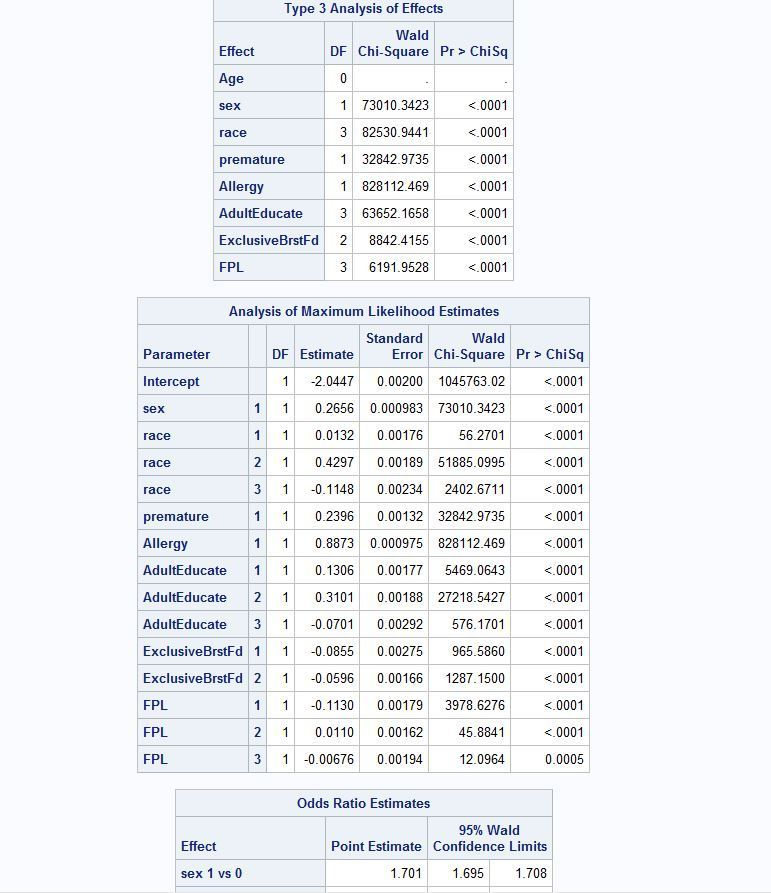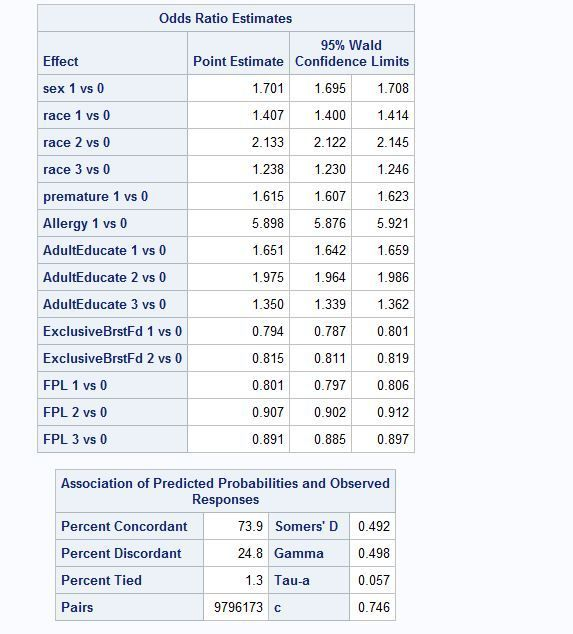## Age as categorical Logistic regression

Please i will appreciate if someone can help explain what happened here.

I ran an adjusted model to get the Odds Ratio for these variables and i could not find the OR for age :

proc surveylogistic data = second1;
weight fwc;
class sex (ref = '0') race(Ref = '0')age (ref= '0') premature(ref= '0') smokeInside (Ref= '0')
allergy(Ref = '0') AdultEducate (Ref = '0') ExclusiveBrstfd(Ref = '0') FPL (Ref='0');
Model asthma (event ='1') = age sex race premature allergy AdultEducate ExclusiveBrstFd FPL;
run;

In my initial Bivariate analysis, i got an OR for age.

I coded age groups "0-5", "6-11" "12-17" as 0, 1 and 2 respectively with 0 as my reference group.

Please can anyone explain what happened here?

Attached is the output for the multivariate analysis without age.1 ACCEPTED SOLUTION

Accepted Solutions

## Re: Age as categorical Logistic regression

Show all the output, specifically, check the number of observations used as well as the design matrix.
6 REPLIES 6

## Re: Age as categorical Logistic regression

Does your log show any notes/warnings?

## Re: Age as categorical Logistic regression

NO. The Log did not show any warnings.

## Re: Age as categorical Logistic regression

Show all the output, specifically, check the number of observations used as well as the design matrix.

## Re: Age as categorical Logistic regression

Use PROC FREQ to doublecheck the levels of the AGE variable. Is it possible that AGE is continuous and that you named the recoded (binned) variable something else?

## Re: Age as categorical Logistic regression

It may be a good idea to show HOW you created your age categories.

I have seen this behavior when someone uses code similar to

data have;

set have;

if age in (1 2 3 4 5) then age=0;

<similar for the other age groups>

run;

And for some reason ran that data step more than once, perhaps adding other recodes. The first pass would reduce the age range to 0,1,2. The second would then turn the 1 and 2 "groups" to the 0 group. So all values of "age" are now 0 and with one value 0 DF

## Re: Age as categorical Logistic regression

Thank you very much.

Discussion stats
• 6 replies
• 1270 views
• 2 likes
• 5 in conversation